# rhodonea

## roulette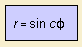Because of its flower-like form, the rhodonea (curve) is also called the rose 1), or the rosette. It is a hypocycloid for which the amplitudes of the two terms of the parameter equation are equal 2). The number of petals is the denominator of: 1/2 - 1/(2c). For irrational c the curve does not close, and the number of petals is infinite. For rational c, the resulting curve is algebraic. Example: for c=5/2 the number of petals is 10 (see below). Some relationships with other curves: the rhodonea is the radial and the pedal of the epicycloid it is also the pedal of the hypocycloid it is the polar inverse of the epi spiral the conchoid of the curve is the botanic curve Some authors confine the constant c to integer values. In that case the number of petals is: c (for odd c) 2c (for even c) The degree of the Cartesian equation of the curve is then: c + 1 (for odd c) 2 (c + 1) (for even c)Some integer rhodonea curves are the following: The circle is the rose with c=1. For c=3, the (regular) trifolium 3), the three-leaved rose, or three-leaved clover, a quartic curve 4), is the radial of the deltoid. I add the regular prefix in the name to distinguish the curve from the more general trifolium.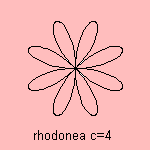For c=2, the quadrifolium 5), the four-leaved rose, a sextic curve 6), has a remarkable relationship with the astroid: the curve is the radial, the pedal and the orthoptic of the astroid And its catacaustic (with the source in the center) is also an astroid. A variation on the quadrifolium is the infinite quadrifolium. Next step is to investigate the curves with non-integer values for parameter c: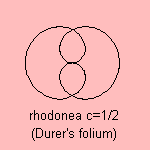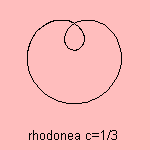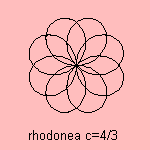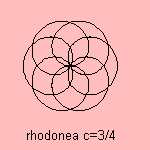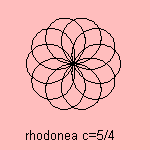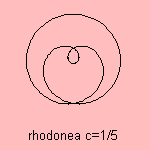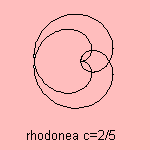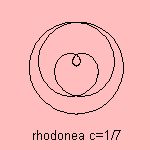For c = 1/2 the rhodonea is called Dürer's folium, after Albrecht Dürer (1471 - 1528). This folium is a sextic 7). The first to investigate the group of rhodonea curves was the Italian mathematician Guido Grandi (1723), an Italian priest, member of the order of the Camaldolites. He was professor in Mathematics at the University of Pisa. Therefore the curve has also the name of rose of Grandi. Johan Gielis extended the rose to a super rose. notes 1) Rhodon = rose. 2) The result is a formula in which the radius is a cosine function of the rolled angle (which is proportional to the polar angle). The relation between the rhodonea's parameter c and the parameters a and b of the hypotrochoid is: 1/c = 1 - 2 a, where b = 1/a - 1. 3) Tres (Lat.) = three, folium (Lat.) = leaf. 4) In Cartesian coordinates: (x2 + y2)2 = x ( x2 - 3y2). 5) Quattuor (Lat.) = four, folium (Lat.) = leaf. 6) Its Cartesian equation is the following: (x2+y2)3 = x2y2 7) Its Cartesian equation is the following: (x2+y2) (2(x2+y2)-1)2 = x2.